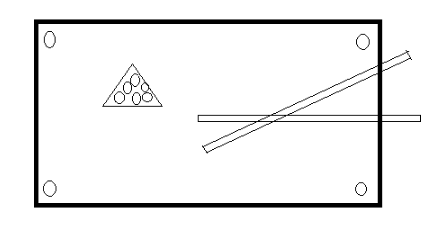Shown below is the top view of a snooker table.The area of the table is $28$ sq. m. and its length is $7$ m.What is the width of the snooker table?A. $4\;m$ B. $7\;m$ C. $8\;m$ D. $14\;m$Verified
123.3k+ views
Hint: We know that the snooker is in the shape of the rectangle and area of the rectangle can be given by the product of length and the width. Here we will place the value of the area and the length and will find the value for the width for the resultant answer.

Given that the area of the table is $= 28$ sq. m.
Also, given that the length of the table is $= 7$ m
Let the width of the table be $= w$
Now, the area of the rectangular snooker table is $=$ length $\times$ Width
Place the values in the above equation –
$\Rightarrow 28 = 7 \times w$
The above equation can be re-written as –
$\Rightarrow 7 \times w = 28$
The term multiplication on one side is moved to the opposite side then it goes to the denominator.
$\Rightarrow w = \dfrac{{28}}{7}$
Find the factors of the term in the numerator on the left hand side of the equation.
$\Rightarrow w = \dfrac{{7 \times 4}}{7}$
Common factors from the numerator and the denominator cancel each other. Therefore remove from the numerator and the denominator.
$\Rightarrow w = 4\;m$
Hence, from the given multiple choices – the option A is the correct answer.
So, the correct answer is “Option A”.

Note: Do not forget to write the appropriate units after the solution. Know the difference between the area and circumference of the rectangle. Area is represented in square units and it is the product of two adjacent sides whereas the circumference is the sum of all the four sides of the rectangle.